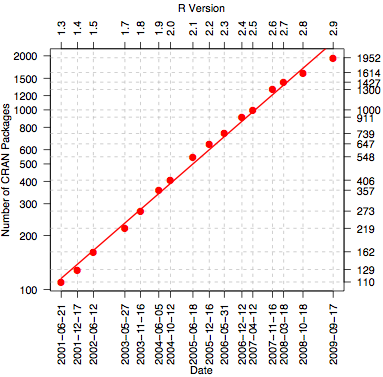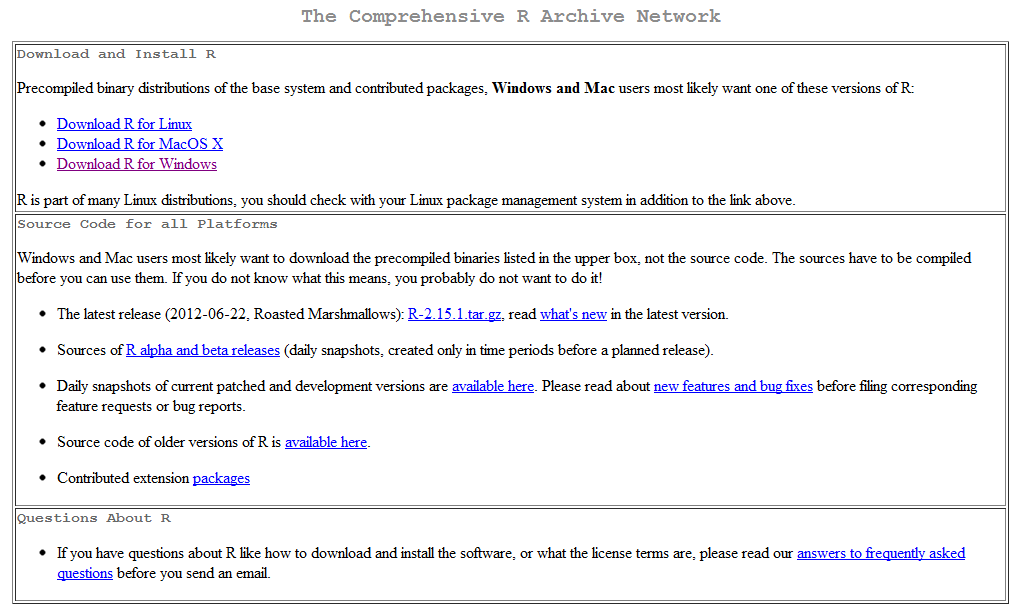# So Many Packages!# CRAN’s Home• RStudio
• devtools
• roxygen
• Git
• MingW

# Folders

 R R Code man Help Files src Compiled code such as C/C++/FORTRAN data Included Data inst Files to be included in binary

# Files

 DESCRIPTION NAMESPACE NEWS LISCENSE README Package information including dependencies List of functions exposed to end user What has been updated in each version Copyright information Basic description of package

# DESCRIPTION

 Package Name of package Type Just use Package Title Verbose Title Version Current version: v.s.ss Date Latest build date Author Name of author Maintainer Author email address: name at domain dot com Description Short description of package License License type LazyLoad Just use yes Depends Comma separated list of packages to be loaded Imports Comma separated list of packages to use but not load Suggests Comma separated list of packages that are nice to have Collate List (no commas) of r files in the R directory in processing order ByteCompile If the package should be Byte Compiled on installation

# Example DESCRIPTION

Package: coefplot
Type: Package
Title: Plots Coefficients from Fitted Models
Version: 1.1.7
Date: 2011-10-02
Author: Jared P. Lander
Maintainer:
Description: Plots the coefficients from a model object
Depends: ggplot2
Imports: plyr, stringr, reshape2, useful
Collate: coefplot.r, modelInfo.r, utilities.r, coefplot-package.r, multiplot.r
ByteCompile: TRUE

# NAMESPACE

 import Package to use export Function for end user S3method Identify generic methods

# NAMESPACE Example

S3method(coefplot,lm)
S3method(coefplot,rxLinMod)
S3method(coefplot,rxLogit)
S3method(getModelInfo,lm)
S3method(getModelInfo,rxLinMod)
S3method(getModelInfo,rxLogit)
export(coefplot)
export(coefplot.lm)
export(coefplot.rxLinMod)
export(coefplot.rxLogit)
export(multiplot)
export(plotcoef)
import(ggplot2)
import(plyr)
import(reshape2)
import(stringr)
import(useful)

# NEWS Example

Version 1.1.7
Thanks to Felipe Carrillo I have fixed a bug in multiplot. Previously, if multiple models with the same formula but different data.frames were inputed then they would all have the same name (even if specified with the names argument) and only one model would be plotted. This now works as expected, plotting all the models regardless of identical formulas.

Version 1.1.6
Made change to reshape2::melt so that variable.name and value.name behave properly with new ggplot2

Version 1.1.5
Fixed glitch that didn’t plot inner CI for single plots or multipane multiplots

Coefplot is a package for plotting the coefficients and standard errors from a variety of models. Currently lm, glm, rxLinMod and rxLogit are supported.

The package is designed for S3 dispatch from the functions coefplot and getModelInfo to make for easy additions of new models.

# Documenting Example

#' simple.ex
#' Simple Example
#' This is a simple example of a function
#' @aliases simple.ex
#' @author Jared P. Lander
#' @export simple.ex
#' @param x A numeric
#' @param y A second numeric
#' @return x times y
#' @examples
#' simple.ex(5, 3)
simple.ex <- function(x, y)
{
return(x * y)
}

# The Help File

\name{simple.ex}
\alias{simple.ex}
\title{within.distance}
\usage{simeple.ex(x, y)}
\arguments{
\item{x}{A numeric}
\item{y}{A second numeric}
}
\value{x times y}
\description{Compute distance threshold}
\details{This is a simple example of a function}
\author{Jared P. Lander}
\examples{
simple.ex(3, 5)
}

# S3 Methods

#' print.myClass
#' @aliases print.myClass
#' @method print myClass
#' @S3method print myClass
#' @export print.myClass
#' @param x Simple object
#' @param \dots Fi=urther arguments to be passed on
#' @return The top 5 rows of \code{x}
print.myClass <- function(x, ...)
{
class(x) <- "list"
x <- as.data.frame(x)
}

require(devtools)
document(pkg = "pkg", clean = TRUE)
## Writing simple.ex.Rd
## Writing simple.helper.Rd
## Writing print.myClass.Rd

Linux/Mac

$R CMD build simple Windows # Create tar$ R CMD build simple
# create zip
$R CMD INSTALL --build simple Devtools build(pkg = "pkg", binary = TRUE) # Check Your Package Linux/Mac/Windows $ R CMD check simple_1.0.0.tar.gz

Devtools

check(pkg = "pkg")

Warnings and Errors must be fixed before submitting to CRAN

• Email ligges<at>statistik<dot>tu-dortmund<dot>de
and
cran<at>r-project<dot>org

• with CRAN Upload: simple 1.0.0 in the subject

Use it

# The R Function

#' vect.add
#' @useDynLib simple
{
.Call("vector_add", x, y, PACKAGE = "simple")
}

# The C++ Function

#include <Rcpp.h>
using namespace Rcpp;

RcppExport SEXP vector_add(SEXP x, SEXP y)
{
NumericVector x_(x); NumericVector y_(y);
NumericVector result(x_.size());
// for each element add the two cells
for(int i=0; i<x_.size(); ++i)
{
result[i] = x_[i] + y_[i];
}

return(wrap(result));
}

# Simpler C++

#include <Rcpp.h>
using namespace Rcpp;

RcppExport SEXP vector_add(SEXP x, SEXP y)
{
NumericVector x_(x); NumericVector y_(y);

return(wrap(x_ + y_));
}

# Building

Same commands as with plain R

# You need…

• Proper Folder Structure
• Proper Files
• Documentation (with roxygen)
• Build & install from within R
• Publish to CRAN